﻿ C# Operators - TutorialsTrend
This Angular tutorial helps you get started with Angular quickly and effectively through many practical examples.
`

# C# - Operators

An operator performs some operation on single or multiple operands (data value) and produces a result. Operators are symbols or keywords that instruct the C# engine to take certain actions. The addition (+) sign, for example, instructs the C# engine to add two variables or values, whereas the equal-to (=), greater-than (>), or less-than (<) symbols instruct the compiler to compare two variables or values, and so on.

Let us take a simple expression 3 + 5 is equal to 8. Here, 3 and 5 are called operands and "+" is called the operator.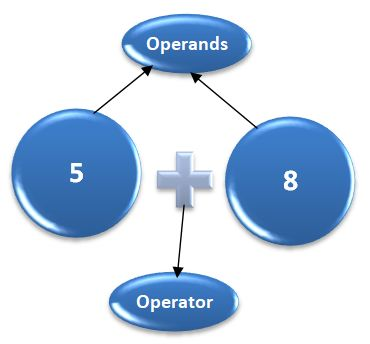C# contains both unary (single operand) and binary operators (two operands).

Unary operator - Operand operator or Operator operand

Example

a++ or ++a

Binary operator -Operand operator operand

Example

a * b

The following operators are available in C#.

1. Arithmetic Operators
2. Assignment Operators
3. Comparison (Relational) Operators
4. Bitwise Operators
5. Logical Operators

#### 1. Arithmetic Operators in C#

Arithmetic Operators are used to perform arithmetic on numbers or operands.

The following table show the list of arithmatic operators in C#.

SNo. Operator Type Description with Example
1 Addition (+) Binary It is used to return the value after addition. ex- (20 + 30)
2 Subtraction (-) Binary It is used to return the value after subtraction. ex- (30 - 20)
3 Multiplication (*) Binary It is used to return the value after multiplying the operands. ex- (30 * 20)
4 Division (/) Binary It is used to return the value after dividing 2 operands. ex- (30 / 3)
5 Remainder (%) Binary It is used to return the integer remainder after dividing 2 operands. ex- (30 % 4)
6 Increment (++) Unary It adds 1 to the operand. For the prefix operator (++var2), it returns the value after adding 1. For the postfix operator (var2++), it adds 1 to the value then returns it. ex- 7++
7 Decrement (--) Unary It subtracts 1 from the operand. For the prefix operator (–var2), it returns the value after subtracting 1. For the postfix operator (var2–), it subtracts 1 from the value then returns it. ex- 7--

You can paste the below code in VisualStudio editor and run the program.

``````using System;

namespace FirstProgram
{
class Program
{
static void Main(string[] args)
{
Console.WriteLine("20 + 30 = " + (20 + 30) );
Console.WriteLine("Subtraction:");
Console.WriteLine("12 - 3 =" + (30 - 20) );
Console.WriteLine("Multiplication:");
Console.WriteLine("12 * 3 =" + (30 * 20) );
Console.WriteLine("Division:");
Console.WriteLine("12 / 3 =" + (30 / 3) );
Console.WriteLine("Remainder:");
Console.WriteLine("12 % 3 =" + (30 % 4) );
var arthvar = 7;
Console.WriteLine("Value of arthvar is " +arthvar);
Console.WriteLine("Increment:");
Console.WriteLine("arthvar++ is " + (arthvar++) );
Console.WriteLine("Value of arthvar is " + arthvar );
Console.WriteLine("++arthvar is " + (++arthvar) );
Console.WriteLine("Value of arthvar is " + arthvar );
Console.WriteLine("Decrement:");
Console.WriteLine("arthvar-- is " + (arthvar--));
Console.WriteLine("Value of arthvar is " + arthvar );
Console.WriteLine("--arthvar is " + (--arthvar));
Console.WriteLine("Value of arthvar is " + arthvar );
}
}
}``````

Output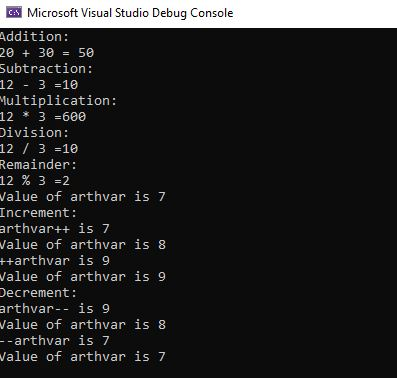#### 2. Assignment Operators in C#

An assignment operator is used to assigns a value to the left operand based on the value of its right operand with the help of equals = sign.

The following table show the list of Assignment operators in C#.

SNo. Operator Description Shorthand Meaning
1 = Assignment x = y x = y
2 += Add and Assignment x += y x = x + y
3 -= Subtract and Assignment x -= y x = x - y
4 *= Multiply and Assignment x *= y x = x * y
5 /= Division Assignment x /= y x = x / y
6 %= Remainder Assignment x %= y x = x % y

You can paste the below code in VisualStudio editor and run the program.

``````using System;
namespace FirstProgram
{
class Program
{
static void Main(string[] args)
{

var a=0;
a = 10;
Console.WriteLine("= Operator =" + a );

a = 20;
a += 30;
Console.WriteLine("+= Operator=" + a );

a = 50;
a -= 20;
Console.WriteLine("-= Operator=" + a );

a = 5;
a *= 25;
Console.WriteLine("*= Operator=" + a );

a = 50;
a /= 10;
Console.WriteLine("/= Operator=" + a );

a = 100;
a %= 15;
Console.WriteLine("%= Operator=" + a);
}
}
}``````

Output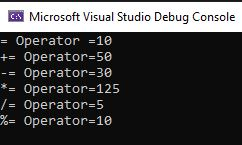#### 3. Comparison Operators

A comparison operator compares its operands and returns a boolean value (true or false) based on whether the comparison is true.

The following table show the list of Comparison operators in C#.

SNo. Operator Name Example Description
1 == Equal x == y True if x is equal to y
!= Not equal x != y True if x is not equal to y
3 < Less than x < y True if x is less than y
4 > Greater than x > y True if x is greater than y
5 >= Greater than or equal to x >= y True if x is greater than or equal to y
6 <= Less than or equal to x <= y True if x is less than or equal to y

You can paste the below code in VisualStudio editor and run the program.

``````using System;

namespace FirstProgram
{
class Program
{
static void Main(string[] args)
{
int x = 35;
int y = 45;
int z = 35;

Console.WriteLine("== Operator : ");
Console.WriteLine(x == z);

Console.WriteLine(" != Operator : ");
Console.WriteLine(x != y);

Console.WriteLine(" < Operator : ");
Console.WriteLine(x < y);

Console.WriteLine(" > Operator : ");
Console.WriteLine(x > y);

Console.WriteLine(" <= Operator : ");
Console.WriteLine(x <= y);

Console.WriteLine(" >= Operator : ");
Console.WriteLine(x >= y);
}
}
}
``````

Output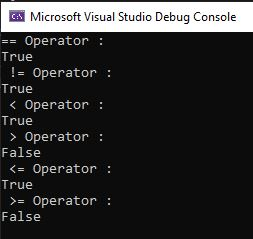#### 4. Bitwise Operators

The bitwise operators perform bitwise operations on operands. The bitwise operators are as follows:

SNo. OperatorNameExampleDescription
1&Bitwise AND(10==20 & 20==33) = 0It returns 1 if both operands are ones
2|Bitwise OR(10==20 | 20==33) = 0It returns 0 if both operands are zero
3^Bitwise XOR(10==20 ^ 20==33) = 0It returns 0 in each bit position for which the corresponding bits are the same and 1 in each bit position for which the corresponding bits are different.
4~Bitwise NOT (~10) = -11The operand's bits are inverted by it.
5<<Bitwise Left Shift(10<<2) = 40It shifts x in binary representation y bits to the left, shifting in zeros from the right.
6>>Bitwise Right Shift(10>>2) = 2It shifts x in binary representation y bits to the right, discarding bits shifted off.

You can paste the below code in VisualStudio editor and run the program.

``````using System;

namespace FirstProgram
{
class Program
{
static void Main(string[] args)
{
Console.WriteLine(10 == 20 & 20 == 33);
Console.WriteLine(10 == 20 | 20 == 33);
Console.WriteLine(10 == 20 ^ 20 == 33);
Console.WriteLine(~10);
Console.WriteLine(10 << 2);
Console.WriteLine(10 >> 2);
}
}
}
``````

Output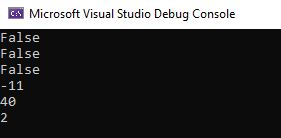#### 5. Logical Operators

These operators are (typically) used with Boolean/ logical values and return a Boolean value. However, if the && and || operators are used with non-Boolean values, they may return a non-Boolean value.

The following table show the list of Logical operators in C#.

SNo.OperatorNameExampleDescription
1 `&&` And `x && y` True if both x and y are true
2 `||` Or `x || y` True if either x or y is true
3 `!` Not `!x` True if x is not true

You can paste the below code in VisualStudio editor and run the program.

``````using System;

namespace FirstProgram
{
class Program
{
static void Main(string[] args)
{
var x = 8;
var y = 3;
Console.WriteLine("Logical AND:");
Console.WriteLine(x < 10 && y > 1);
Console.WriteLine(x < 10 && y < 1);
Console.WriteLine("Logical OR:");
Console.WriteLine(x < 10 || y > 1);
Console.WriteLine(x < 10 || y > 1);
Console.WriteLine(x < 10 || y < 1);
Console.WriteLine("Logical NOT:");
Console.WriteLine(!(x > y));

}
}
}``````

Output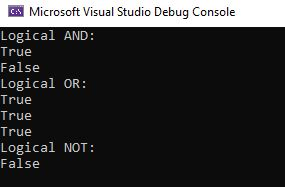Prev Next